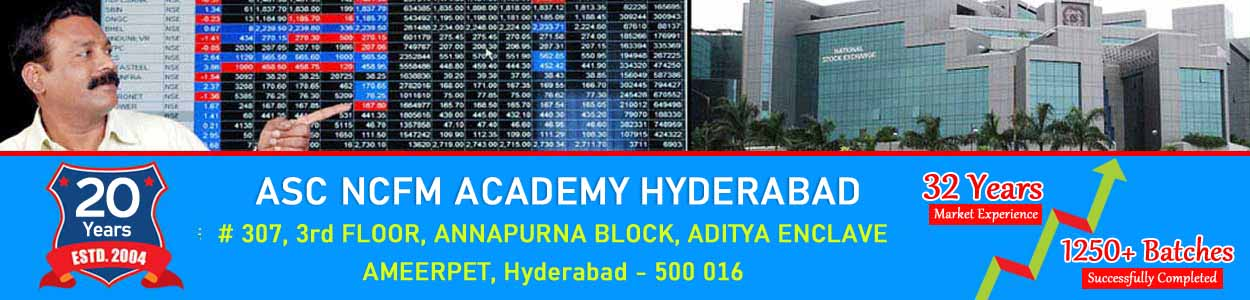# NISM Training Hyderabad : Pricing of Stock Futures : AS Chakravarthy

## AS Chakravarthy NCFM Hyderabad : Stock Market Training InstitutePricing of Stock Futures

A futures contract on a stock gives its holder (Owner) the right and obligation to buy or sell the stocks. Like index futures, stock futures are also cash settled; there is no delivery of the underlying stocks. Just as in the case of index futures, the main differences between commodity and stock futures are that:

• There are no storage costs involved in holding stock.
• Stocks come with a dividend stream, which is a negative cost if you are long the stock and a positive cost if you are short the stock.

Therefore, Cost of carry = Financing cost - Dividends.
Thus, a crucial aspect of dealing with stock futures as opposed to commodity futures is an accurate forecasting of dividends. The better the forecast of dividend offered by a security, the better is the estimate of the futures price.

A. Pricing of stock futures when no dividend expected

The pricing of stock futures is also based on the cost-of-carry model, where the carrying cost is the cost of financing the purchase of the stock, minus the present value of dividends obtained from the stock. If no dividends are expected during the life of the contract, pricing futures on that stock involves multiplying the spot price by the cost of carry. It has been illustrated in the example given below:

XYZ Ltd.’s futures trade on NSE as one, two and three-month contracts.

Money can be borrowed at 10% per annum. What will be the price of a unit of new two-month futures contract on XYZ Ltd. if no dividends are expected during the two-month period?

1. Assume that the spot price of XYZ Ltd. is Rs. 228.
Thus, futures price F = 228e 0.1*(60/365)
= Rs. 231.90

2 Pricing of stock futures when dividends are expected
When dividends are expected during the life of the futures contract, pricing involves reducing the cost of carry to the extent of the dividends. The net carrying cost is the cost of financing the purchase of the stock, minus the present value of dividends obtained from the stock. This is explained in the illustration below:

XYZ Ltd. futures trade on NSE as one, two and three-month contracts. What will be the price of a unit of new two-month futures contract on XYZ Ltd. if dividends are expected during the two-month period?

1. Let us assume that XYZ Ltd. will be declaring a dividend of Rs. 10 per share after 15 days of purchasing the contract.
2. Assume that the market price of XYZ Ltd. is Rs. 140.
3. To calculate the futures price, we need to reduce the cost-of-carry to the extent of dividend received. The amount of dividend received is Rs.10. The dividend is received 15 days later and hence compounded only for the remainder of 45 days.
4. Thus, futures price F = 140e 0.1× (60/365) – 10e 0.1× (45/365) = Rs.132.20